### Fourier Transform of Gaussian *

We wish to Fourier transform the Gaussian wave packet in (momentum) k-space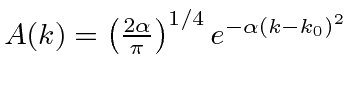to get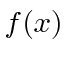in position space. The Fourier Transform formula is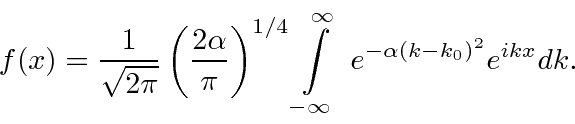Now we will transform the integral a few times to get to the standard definite integral of a Gaussian for which we know the answer. First,which does nothing really since.Now we want to complete the square in the exponent inside the integral. We plan a term likeso we defineAgain. Lets write out the planned exponent to see what we are missing.We need to multiply byto cancel the extra term in the completed square.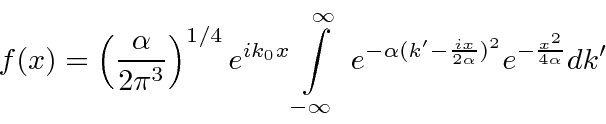That term can be pulled outside the integral since it doesn't depend on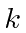.So now we have the standard Gaussian integral which just gives us.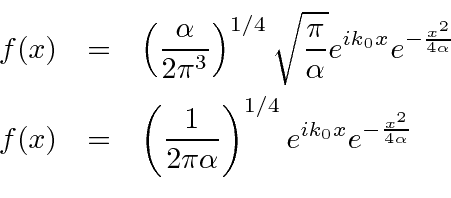Lets check the normalization.Given a normalized, we get a normalized.

The RMS deviation, or standard deviation of a Gaussian can be read from the distribution.Squaring, we get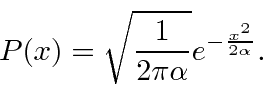Reading from either the coefficient or the exponential we see that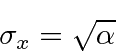For the width in k-space,Reading from the coefficient of the exponential, we get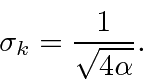We can see that as we vary the width in k-space, the width in x-space varies to keep the product constant.Translating this into momentum, we get the limit of the Heisenberg Uncertainty Principle.In fact the Uncertainty Principle states thatso the Gaussian wave packets seem to saturate the bound!

Jim Branson 2013-04-22